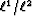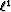Next: Adaptive subtraction of multiples Up: Multidimensional seismic noise attenuation Previous: Multidimensional seismic noise attenuation

## The Huber norm

Chapterintroduces the Huber norm Huber (1973). The Huber norm is an hybriderror measure that is robust to outliers. Because it is differentiable everywhere, the Huber norm can be minimized with a gradient-based algorithm. In Chapter, I propose minimizing the Huber norm with a quasi-Newton method called L-BFGS. This technique can solve any non-linear problem where local-minima are sought. Its limited-memory requirements make it also very attractive for solving large-scale problems. On a velocity estimation problem, this Chapter illustrates that the Huber norm with the L-BFGS solver is robust to outliers, thus providing an alternative to thenorm.Next: Adaptive subtraction of multiples Up: Multidimensional seismic noise attenuation Previous: Multidimensional seismic noise attenuation
Stanford Exploration Project
5/5/2005